# Elastic Net Regression in R Programming

Elastic Net regression is a classification algorithm that overcomes the limitations of the lasso(least absolute shrinkage and selection operator) method which uses a penalty function in its L1 regularization. Elastic Net regression is a hybrid approach that blends both penalizations of the L2 and L1 regularization of lasso and ridge methods.

It finds an estimator in a two-stage procedure i.e first for each fixed λ2 it finds the ridge regression coefficients and then does a lasso regression type shrinkage which does a double amount of shrinkage which eventually leads to increased bias and poor predictions. Rescaling the coefficients of the naive version of the elastic net by multiplying the estimated coefficients by (1 + λ2) is done to improve the prediction performance. Elastic Net regression is used in:

• Metric learning
• Portfolio optimization
• Cancer prognosis

Elastic Net regression always aims at minimizing the following loss function: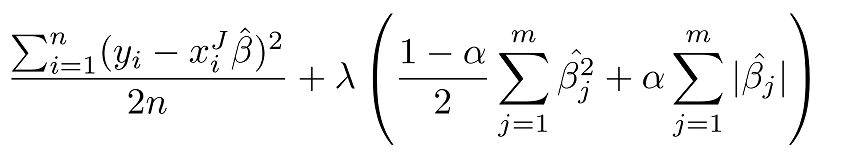Elastic Net also allows us to tune the alpha parameter where alpha = 0 corresponds to Ridge regression and alpha = 1 to Lasso regression. Similarly, when alpha = 0, the penalty function reduces to the L1(ridge) regularization, and when alpha = 1, the penalty function reduces to L2(lasso) regularization. Therefore, we can choose an alpha value between 0 and 1 to optimize the Elastic Net and this will shrink some coefficients and set some to 0 for sparse selection. In Elastic Net regression, the lambda hyper-parameter is mostly and heavily dependent on the alpha hyper-parameter. Now let’s implement elastic net regression in R programming.

### Implementation in R

##### The Dataset

mtcars(motor trend car road test) comprises fuel consumption, performance and 10 aspects of automobile design for 32 automobiles. It comes pre-installed with dplyr package in R.

 `# Installing the package ` `install.packages``(``"dplyr"``) ` `   `  `# Loading package ` `library``(dplyr) ` `   `  `# Summary of dataset in package ` `summary``(mtcars) `

Output: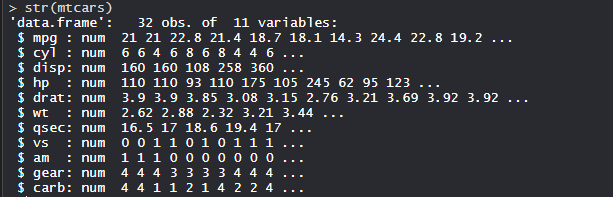##### Performing Elastic Net Regression on Dataset

Using the Elastic Net regression algorithm on the dataset by training the model using features or variables in the dataset.

 `# Installing Packages ` `install.packages``(``"dplyr"``) ` `install.packages``(``"glmnet"``) ` `install.packages``(``"ggplot2"``) ` `install.packages``(``"caret"``) ` ` `  `# X and Y datasets ` `X <- mtcars %>%  ` `     ``select``(disp) %>%  ` `     ``scale``(center = ``TRUE``, scale = ``FALSE``) %>%  ` `     ``as.matrix``() ` `Y <- mtcars %>%  ` `    ``select``(-disp) %>%  ` `    ``as.matrix``() ` ` `  `# Model Building : Elastic Net Regression ` `control <- ``trainControl``(method = ``"repeatedcv"``, ` `                              ``number = 5, ` `                              ``repeats = 5, ` `                              ``search = ``"random"``, ` `                              ``verboseIter = ``TRUE``) ` ` `  `# Training ELastic Net Regression model ` `elastic_model <- ``train``(disp ~ ., ` `                           ``data = ``cbind``(X, Y), ` `                           ``method = ``"glmnet"``, ` `                           ``preProcess = ``c``(``"center"``, ``"scale"``), ` `                           ``tuneLength = 25, ` `                           ``trControl = control) ` ` `  `elastic_model ` ` `  `# Model Prediction ` `x_hat_pre <- ``predict``(elastic_model, Y) ` `x_hat_pre ` ` `  `# Multiple R-squared ` `rsq <- ``cor``(X, x_hat_pre)^2 ` `rsq ` ` `  `# Plot ` `plot``(elastic_model, main = ``"Elastic Net Regression"``) `

Output:

• Training of Elastic Net Regression model: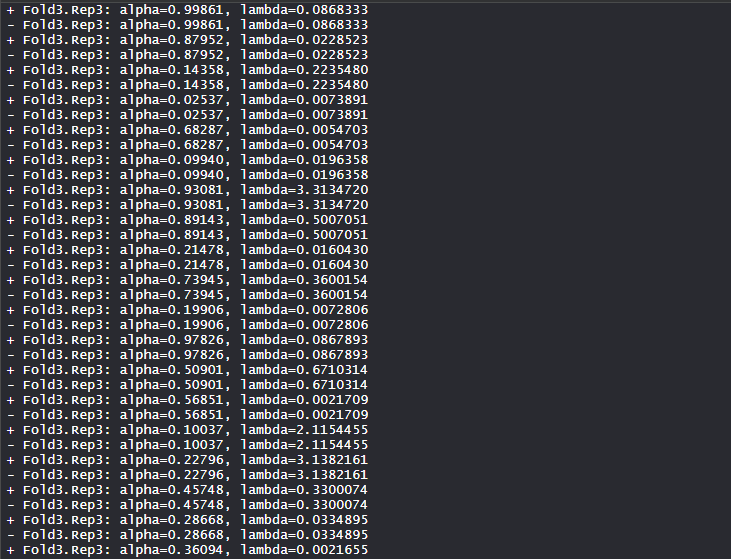The Elastic Net regression model is trained to find the optimum alpha and lambda values.

• Model elastic_model: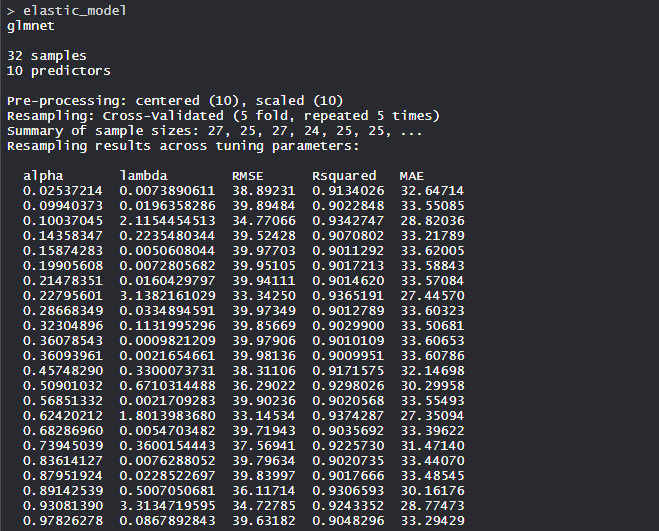The Elastic Net regression model uses the alpha value as 0.6242021 and lambda value as 1.801398. RMSE was used to select the optimal model using the smallest value.

• Model Prediction: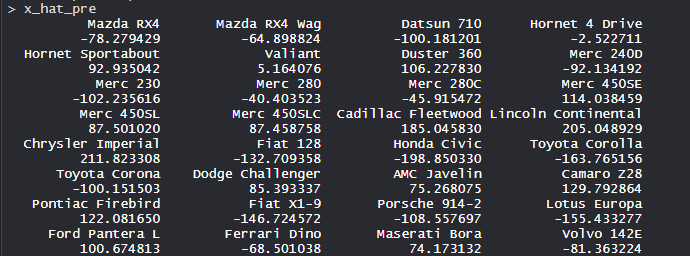The model is predicted using the Y dataset and values are shown.

• Multiple R-Squared: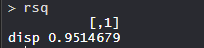The multiple R-Squared values of disp is 0.9514679.

• Plot: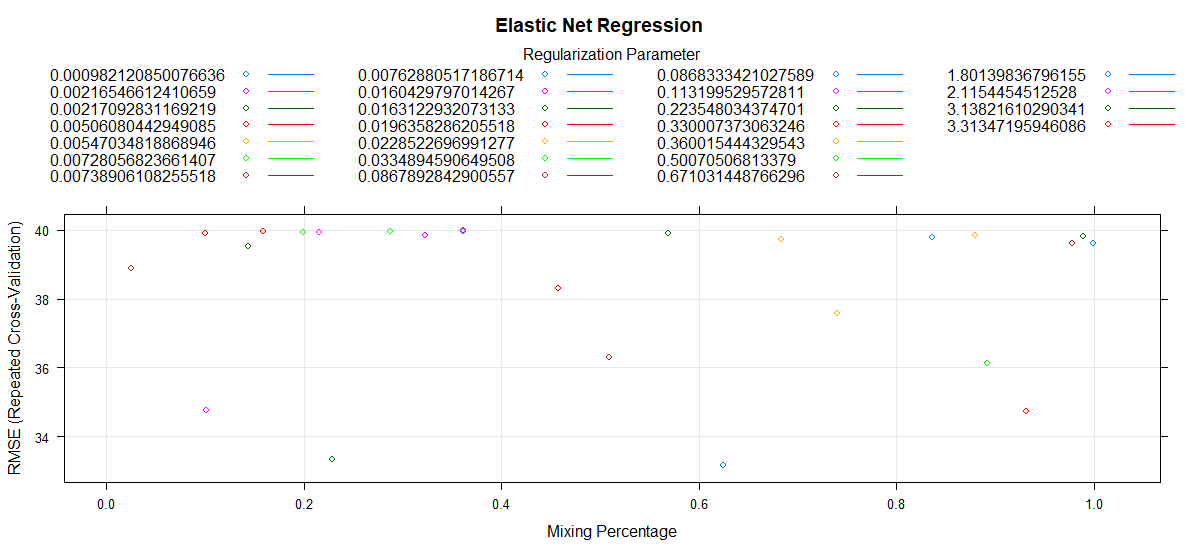The mixing percentage is plotted with RMSE scores with different values of the regularization parameter.

• So, Elastic Net regression applications are used in many sectors of industry and with full capacity.

My Personal Notes arrow_drop_upTechnology Enthusiast

If you like GeeksforGeeks and would like to contribute, you can also write an article using contribute.geeksforgeeks.org or mail your article to contribute@geeksforgeeks.org. See your article appearing on the GeeksforGeeks main page and help other Geeks.

Please Improve this article if you find anything incorrect by clicking on the "Improve Article" button below.

Article Tags :

1

Please write to us at contribute@geeksforgeeks.org to report any issue with the above content.